# Symmetric vs. Skewed Distributions

Brigette Banaszak, Cathryn Jackson
• Author
Brigette Banaszak

Brigette has a BS in Elementary Education and an MS in Gifted and Talented Education, both from the University of Wisconsin. She has taught math in both elementary and middle school, and is certified to teach grades K-8.

• Instructor
Cathryn Jackson

Cat has taught a variety of subjects, including communications, mathematics, and technology. Cat has a master's degree in education and is currently working on her Ph.D.

Explore what symmetric distribution is. Read the difference between skewed and symmetrical data. See how to analyze the symmetric distribution using examples. Updated: 11/06/2021

Show

## Symmetric Distribution Definition

Data is a collection of facts, often numbers such as measurements or statistics. A data set is a group of data collected and often displayed in some sort of visual representation, such as a table or graph. What is meant by the distribution of data? The distribution of data refers to the shape of the data set when displayed on a graph, oftentimes a bar graph. The distribution of a data set can take different shapes, including symmetric and skewed.

What is a symmetric data distribution? A symmetric distribution is a graphic distribution of data that looks nearly the same on both sides. It is important to note that the data does not need to be exactly the same on both sides to be considered symmetric, only nearly the same. Think of a mirror in the middle of the data distribution. Both sides should be near mirror images of one another.

Below is an example of a symmetric data distribution, shown as a symmetric bar graph. The graph shows the number of questions answered correctly by a fictional class of students on a pop quiz. The scores, in order from lowest to highest, are 0, 1, 1, 2, 2, 2, 3, 3, 3, 3, 4, 4, 4, 4, 4, 5, 5, 5, 5, 5, 5, 6, 6, 6, 6, 6, 7, 7, 7, 7, 8, 8, 8, 9, 9, 10.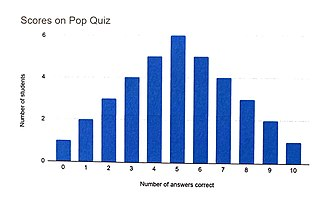The two sides of the graph mirror, or nearly mirror, one another. The peak of the graph is right in the middle. An equal number of students scored above and below the peak.

An important thing to know about a symmetric data set is that the mean, median, and mode will all occur at the same point. The mean, or average, the median, or exact middle number, and the mode, or most common number, will all be the same in a symmetric data set.

Consider again the bar graph of the pop quiz results, which is shown above. The mean, median, and mode of the data set all occur at the number 5, making this a symmetric distribution.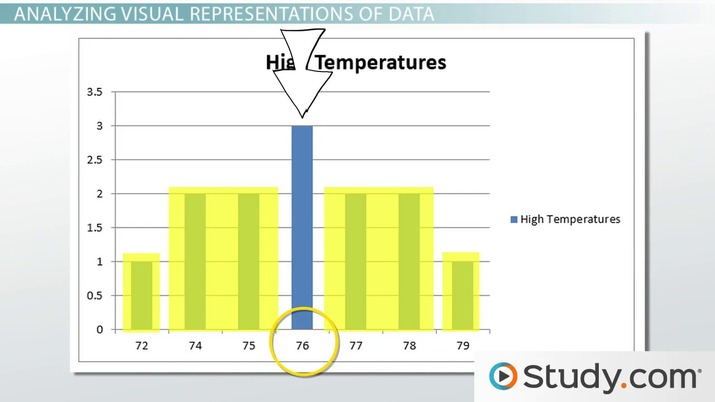An error occurred trying to load this video.

Try refreshing the page, or contact customer support.

Coming up next: Unimodal & Bimodal Distributions: Definition & Examples

### You're on a roll. Keep up the good work!

Replay
Your next lesson will play in 10 seconds
• 0:02 Visual Representations of Data
• 2:17 Analyzing Visual…
• 3:59 Lesson Summary
Save Save

Want to watch this again later?

Timeline
Autoplay
Autoplay
Speed Speed

## Skewed Distribution Definition

What is a skewed data distribution? A skewed distribution is not symmetric, but instead peaks on one side or the other. A distribution might be very skewed or only slightly skewed. Consider the examples below.

The graph below, in red, shows an example of a skewed distribution of data. The two sides of the graph do not mirror one another, and the peak is not in the middle. There are many more data points on the right side of the graph. This graph shows the number of questions answered correctly by a fictional class of students on an announced quiz. There are many more high-scoring students than low-scoring students. The mean, median, and mode of this data set will not occur at the same number. A graph of a distribution such as this, that has a peak on the right and a tail to the left, is said to be skewed left. If the peak of the data distribution is on the left side and the tail is on the right, the distribution is skewed right.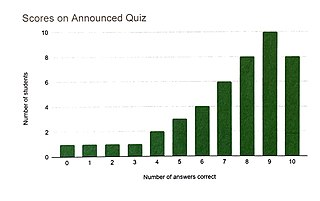The graph below shows the quiz scores of a fictional class in which the quizzes were graded with the wrong answer key. This distribution is not symmetric, but it is instead skewed right. Notice also the empty spaces for scores of 7 or 8 answers correct. An area such as this, with no data points or observations, is called a gap.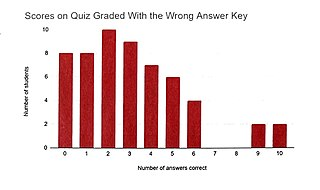The graph below shows the quiz scores of a fictional class on a quiz. This data distribution is neither symmetric nor skewed, but it is distributed in a more random way. Notice that this graph has two peaks: one at a score of 3 and the other at a score of 8. This means the data set has two modes. A distribution such as this is said to be bimodal, which means it has two modes.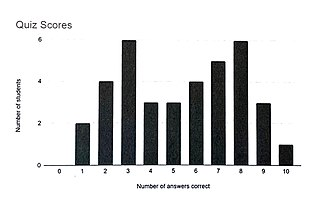### Skewed and Symmetric Distribution Examples

What are examples of distributions that might be symmetric or skewed? Any distribution can take on either shape, but some are more likely than others to be usually symmetric or usually skewed. Distributions that tend to be symmetric include standardized test scores, heights of adult men or adult women, weather data over a long period of time, and salaries. In these cases, the peak is likely to be in the middle, and the two sides of the graph are likely to be nearly symmetric. Some distributions tend to be skewed. An example of this is the number of adults living in a household. Most households will have 1, 2, or perhaps 3 adults, and few will have 6, 7, or 8 adults. Such a data distribution is likely to have a peak on the left and a tail on the right, and it will be considered skewed right.

## Graphing Data Sets

In a visual representation of a data set, the distribution can easily be seen. Common visual representations used to display data sets and their distributions are bar graphs, histograms, dot plots, line plots, and box and whisker plots.

Bar graphs and histograms are very similar, but a bar graph shows one value per bar, while a histogram shows a range of values per bar. The graphs shown at the beginning of the lesson are bar graphs, and the one shown below is a histogram. The histogram shows a distribution of iris petals of varying lengths. Each interval is marked as 1 centimeter, and there are 2 bars in each interval. The first bar in the first interval shows the number of petals between 0 and 0.49 centimeters, and the second bar shows the number of petals between 0.5 and 0.99 centimeters. This is how a histogram is able to show a range of values.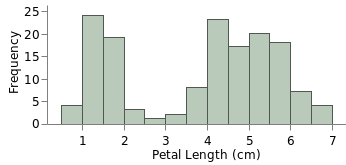A dot plot uses points rather than bars to show the distribution of data. A random dot plot is shown below. A line plot uses x's rather than dots.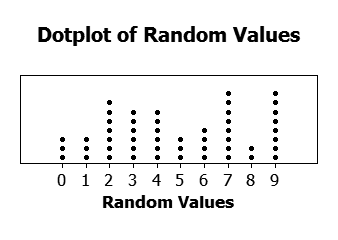A box and whisker plot uses a box and a whisker to show each side of the distribution.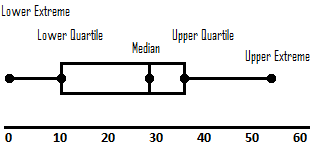To unlock this lesson you must be a Study.com Member.

#### How do you tell if a bar graph is skewed right or left?

A graph with a peak on the right and a tail that extends to the left is said to be skewed left.

A graph with a peak on the left and a tail that extends to the right is said to be skewed right.

#### How do you know if data is symmetric?

A set of data is symmetric if the mean, median, and mode all occur at the same number. When graphed, the two sides of the graph will be almost mirror images of one another.

#### What is an example of a symmetrical distribution?

Standardized test scores are an example of a symmetrical distribution. The mean, median, and mode of the data set will all occur at the same value.

### Register to view this lesson

Are you a student or a teacher?